Go back to  'Geometry'

Explore the world of quadrilaterals and their properties by going through its various aspects. Find answers to questions like what are quadrilaterals, how they are formed, easy ways to identify them, and commonly seen quadrilaterals around us. Become a champ by playing around with interactive simulations and solving interesting questions on quadrilaterals.

A quadrilateral is a closed shape that is obtained by joining four points among which any three points are non-collinear.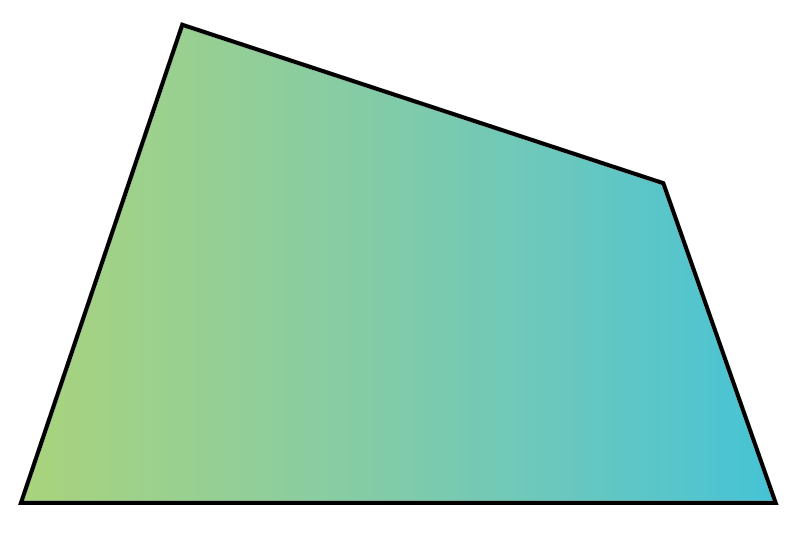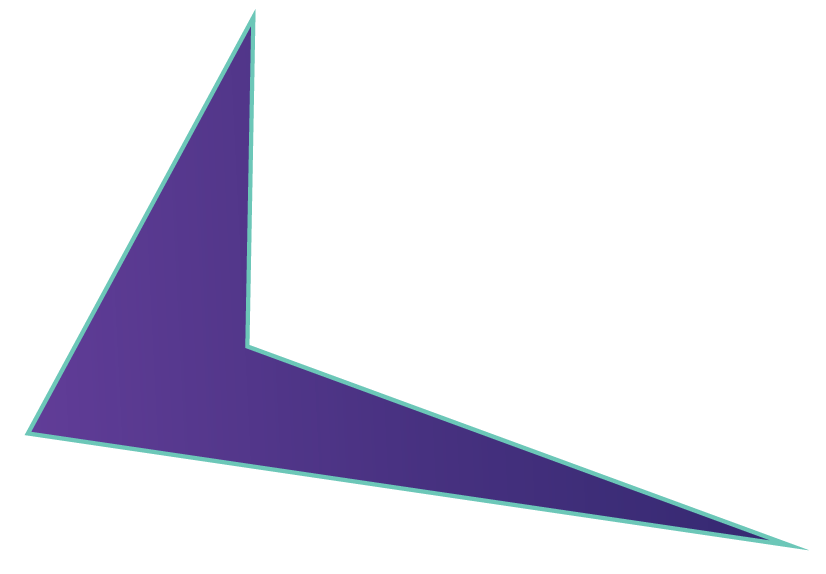We can observe that a quadrilateral has four sides, four angles, and four vertices.

When we have to name a quadrilateral, we have to keep in mind the order of the vertices.

For example, consider the following quadrilateral: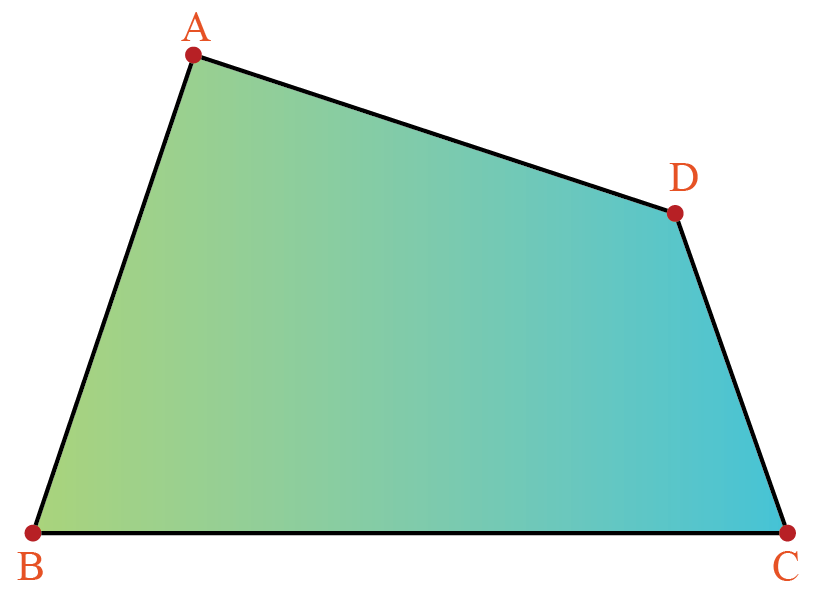We could name this quadrilateral $$ABCD, BCDA, ADCB, DCBA$$, etc, but we cannot name it $$ACBD, BACD$$, etc. since in the latter names, the order of the vertices is wrong.

A quadrilateral ABCD will have two diagonals: $$AC$$ and $$BD$$.

There are different types of quadrilaterals.

Here are a few types of quadrilaterals:

1 Square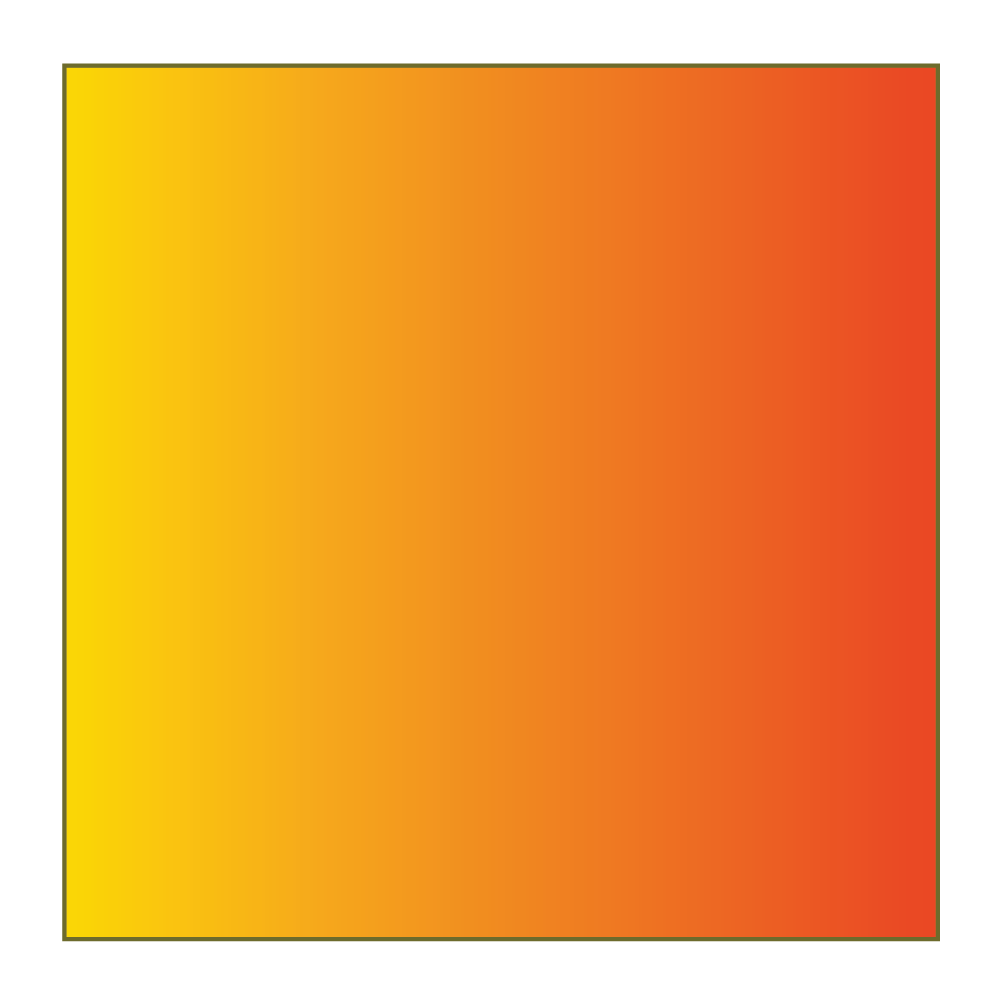2 Rectangle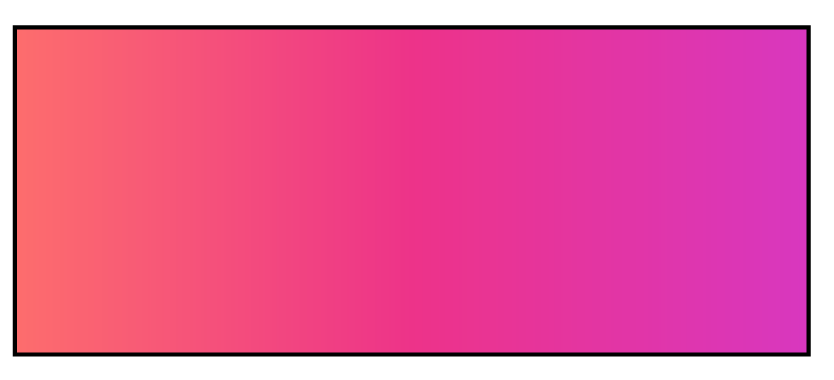3 Parallelogram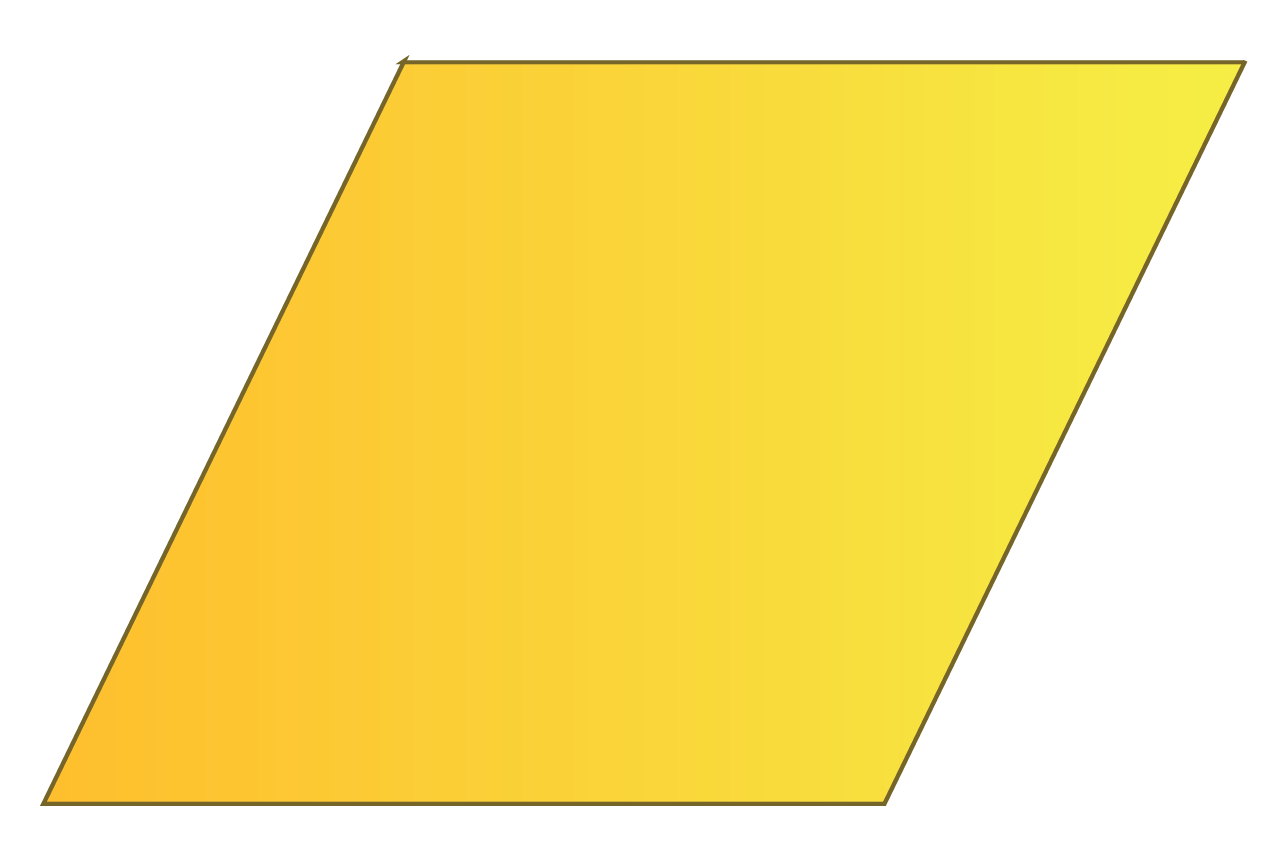4 Trapezium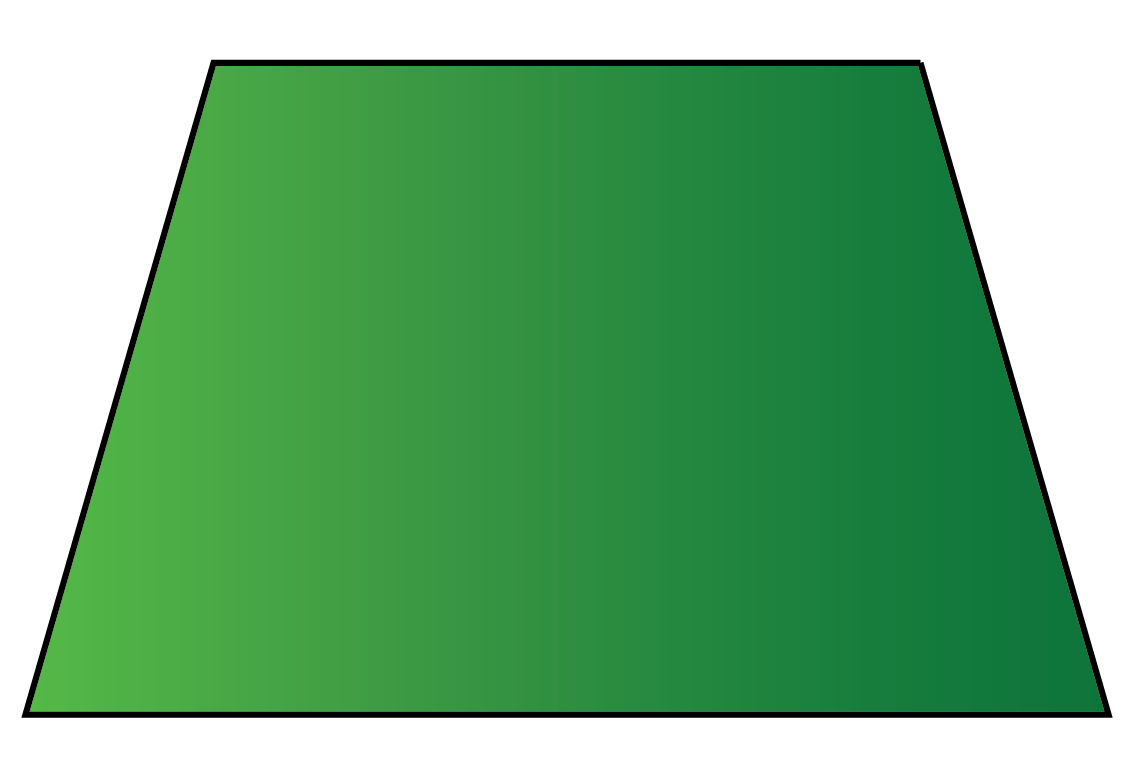5 Rhombus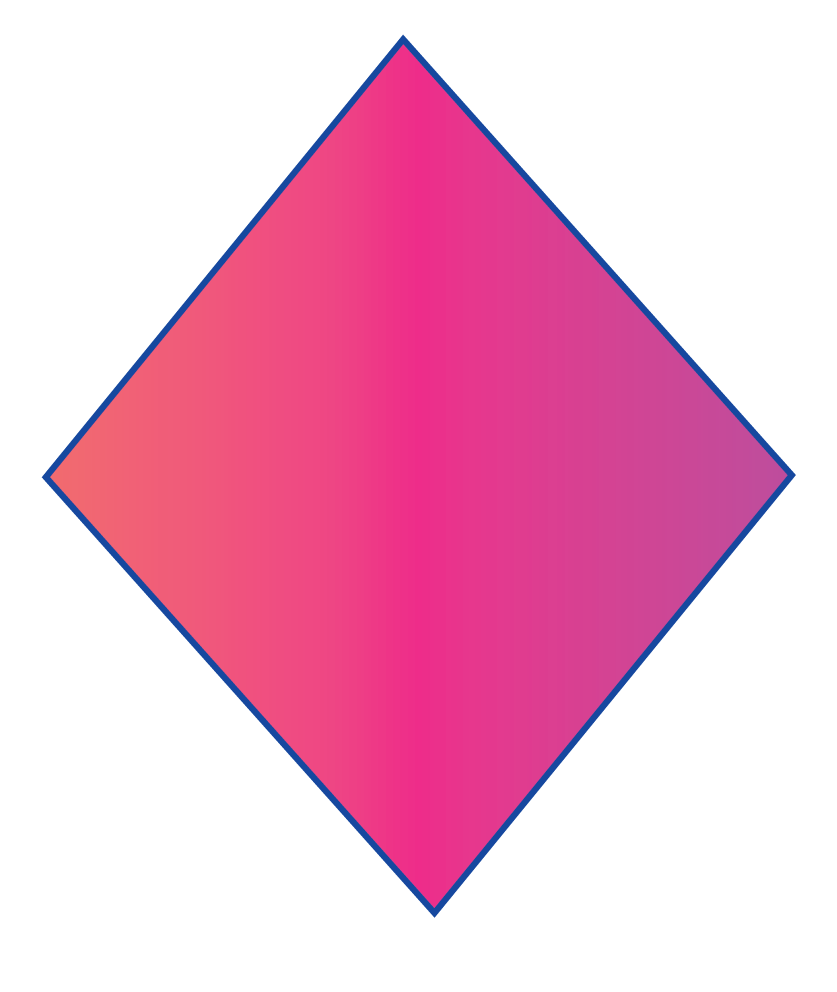6 Kite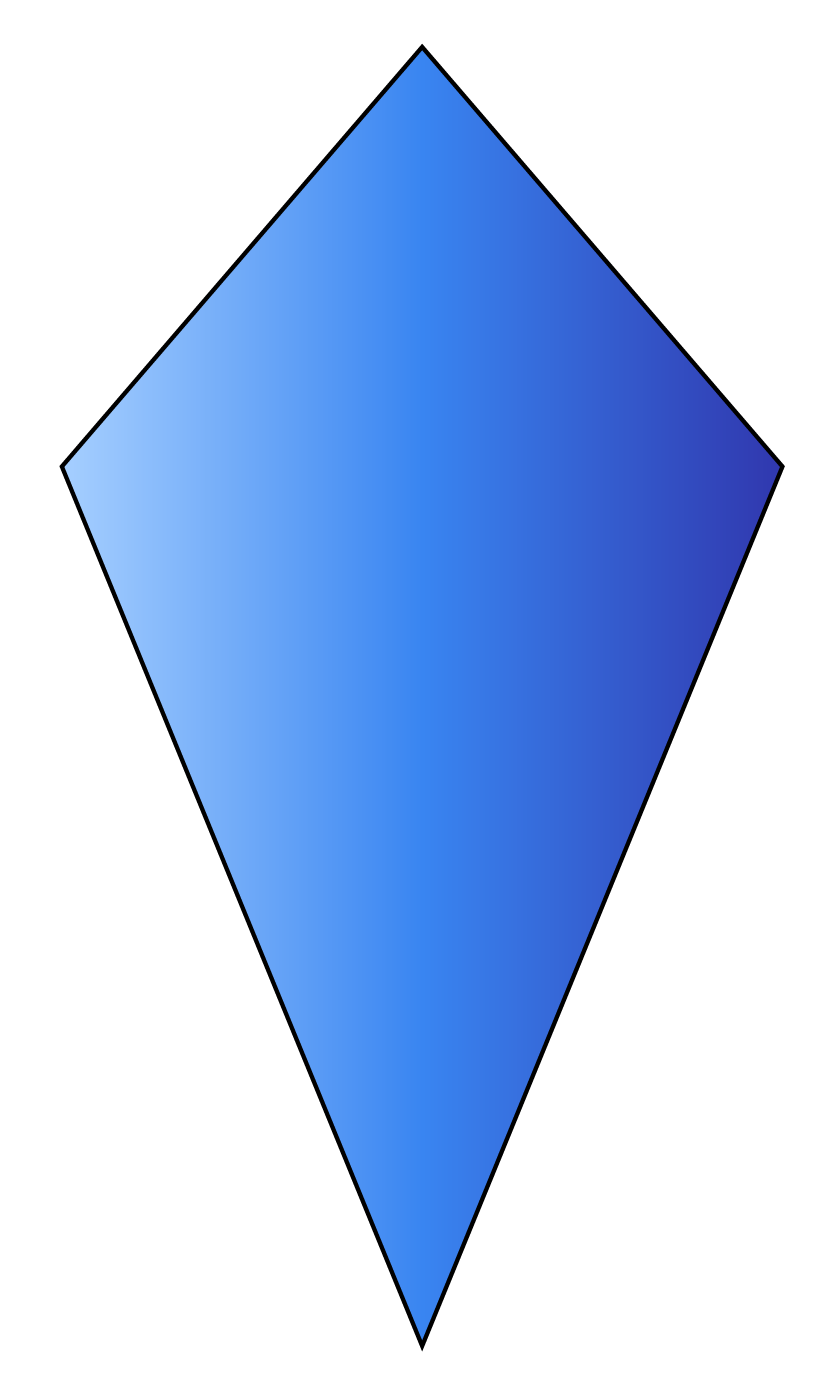The common properties of quadrilaterals are:

• They have four sides.
• They have four vertices.
• They have two diagonals.
• The sum of all interior angles is $$360^\circ$$.

We will study the other properties of different quadrilaterals in detail.

We can identify a quadrilateral by using the following properties of quadrilaterals.

### Square

A square is a quadrilateral with four equal sides and four right angles.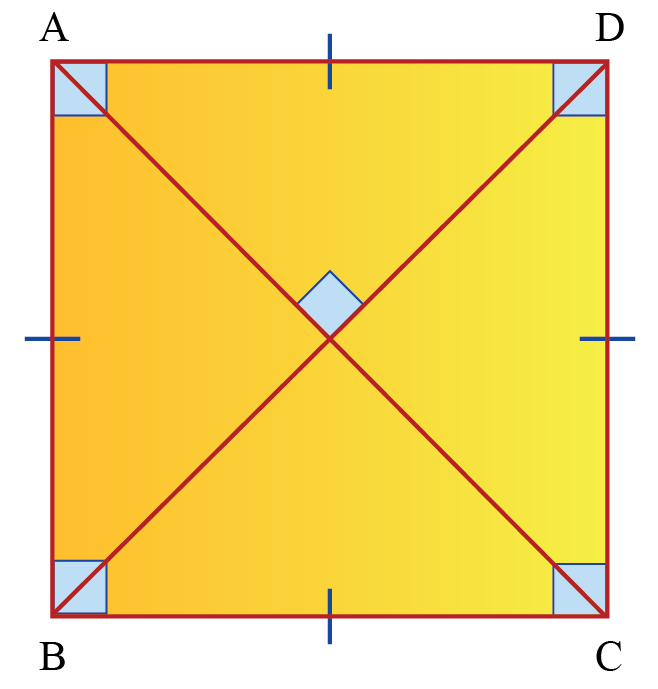A square has:

• four equal sides
$AB=BC=CD=DA$
• four right angles
$\angle A=\angle B=\angle C=\angle D=90^{\circ}$
• two pairs of parallel sides
$A B\|D C \text { and } A D\| B C$
• two equal diagonals
$AC=BD$
• diagonals that are perpendicular to each other
$AC \perp BD$
• diagonals that bisect each other. i.e., one diagonal divides the other diagonal into exactly two halves.

### Rectangle

A rectangle is a quadrilateral with two pairs of equal and parallel opposite sides and four right angles.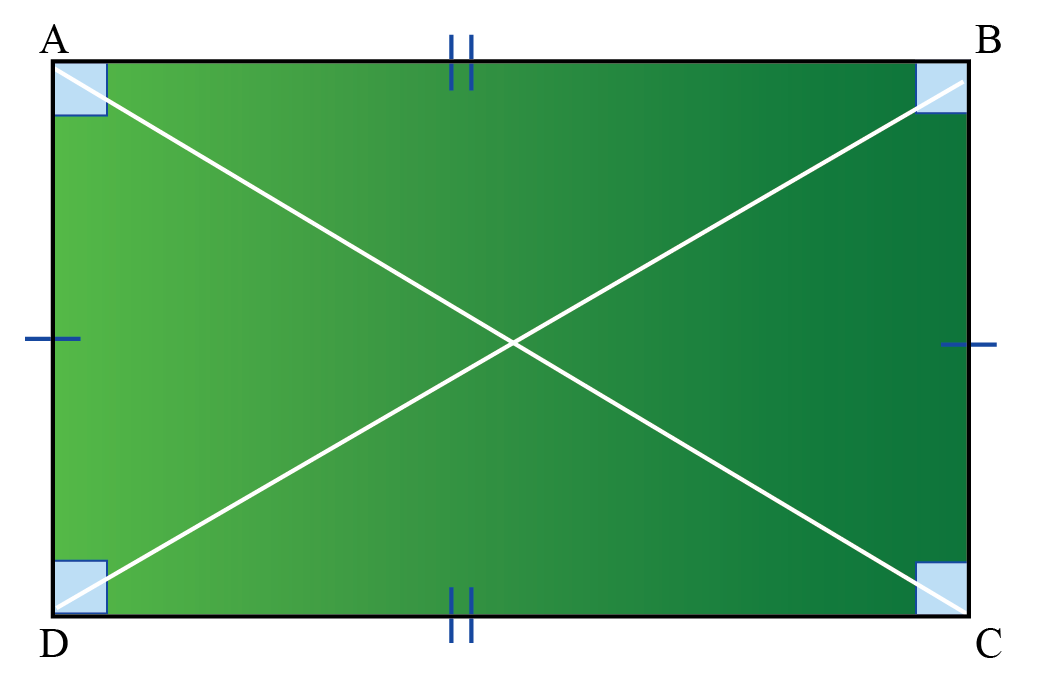A rectangle has:

• two pairs of parallel sides
$A B\|D C \text{ and } A D\| B C$
• four right angles
$\angle A=\angle B=\angle C=\angle D=90^{\circ}$
• opposite sides of equal lengths
$A B=D C \text{ and } A D= B C$
• two equal diagonals
$AC=BD$
• diagonals that bisect each other. i.e., one diagonal divides the other diagonal into exactly two halves.

### Parallelogram

A parallelogram is a quadrilateral in which both pairs of opposite sides are parallel.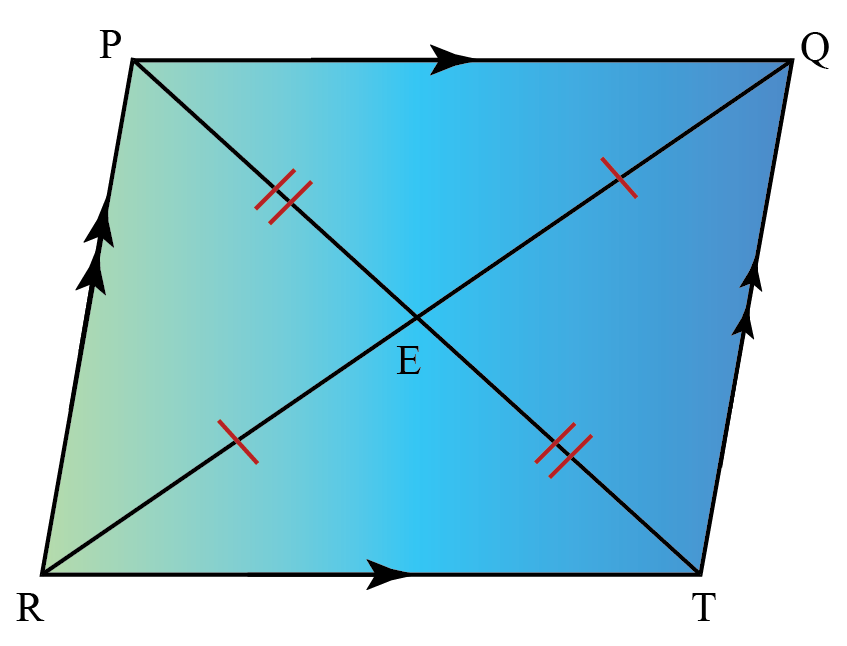A parallelogram has:

• two pairs of parallel sides
$P Q\|R T \text{ and } PR\| Q T$
• opposite sides of equal lengths
$P Q=R T \text{ and } PR= Q T$
• opposite angles that are equal
$\angle P = \angle T \text{ and }\angle Q = \angle R$
• two diagonals that bisect each other. i.e., one diagonal divides the other diagonal into exactly two halves.

### Trapezium

A trapezium is a quadrilateral in which one pair of opposite sides is parallel.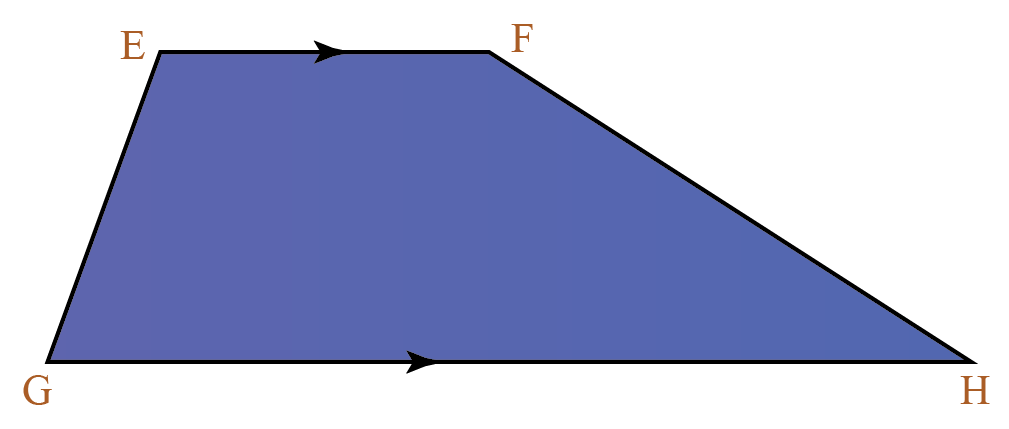• The sides that are parallel to each other are called bases.
In the above figure, $$EF$$ and $$GH$$ are bases.

• The sides that are not parallel to each other are called legs.
In the above figure, $$EG$$ and $$FH$$ are legs.

There is nothing special about the sides, angles, or diagonals of a trapezium.

But if the two non-parallel opposite sides are of equal length, then it is called an isosceles trapezium.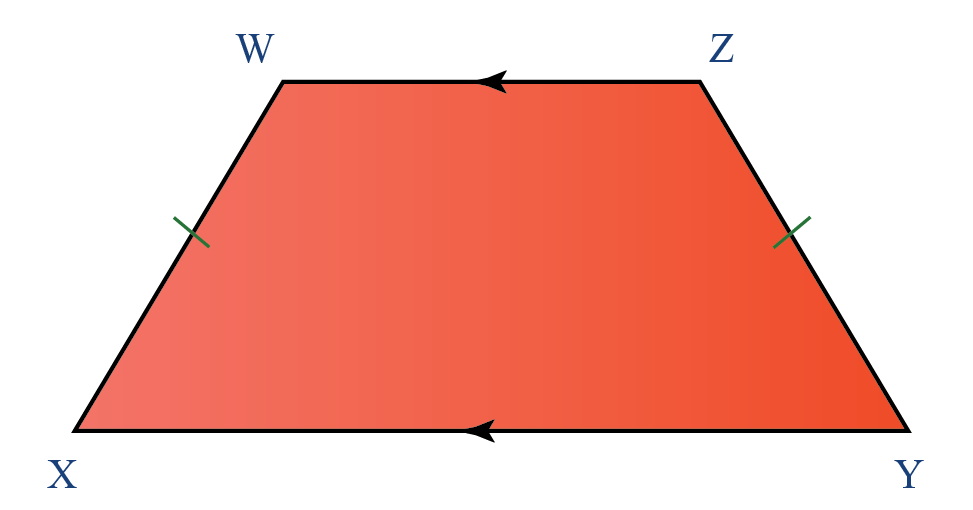The above quadrilateral $$XYZW$$ is an isosceles trapezium.

In an isosceles trapezium, the lengths of the diagonals are equal. i.e., $$XZ=WY$$.

### Rhombus

A rhombus is a quadrilateral with four equal sides.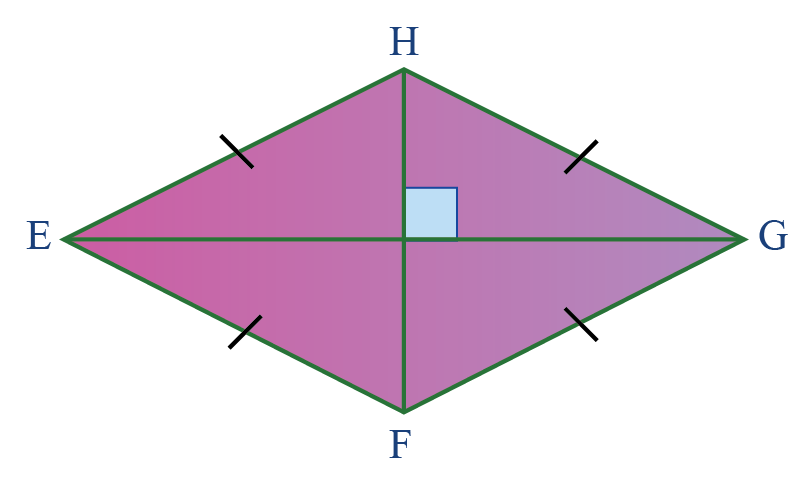A rhombus has:

• two pairs of parallel sides
$E H\|F G \text{ and } E F\| H G$
• four equal sides
$EH=HG=GF=FE$
• opposite angles are equal
$\angle E = \angle G \text{ and } \angle H = \angle F$
• diagonals that are perpendicular to each other
$EG \perp HF$
• diagonals that bisect each other. i.e., one diagonal divides the other diagonal into exactly two halves.

### Kite

A kite is a quadrilateral in which two pairs of adjacent sides are equal.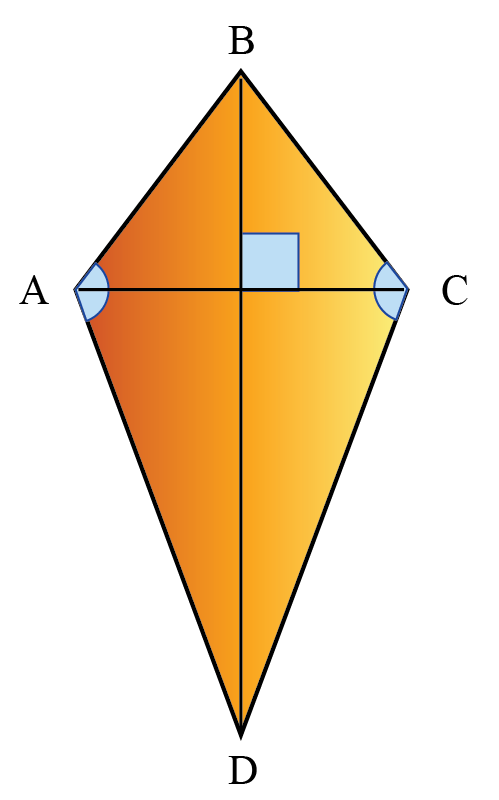A kite has:

• two pairs of equal adjacent sides
$AB=BC \text{ and }CD=DA$
• one pair of opposite angles (which are obtuse) that are equal
$\angle A = \angle C$
• diagonals that are perpendicular to each other
$AC \perp BD$
• a longer diagonal that bisects the shorter diagonal.

We can understand the hierarchy of quadrilaterals using the following chart.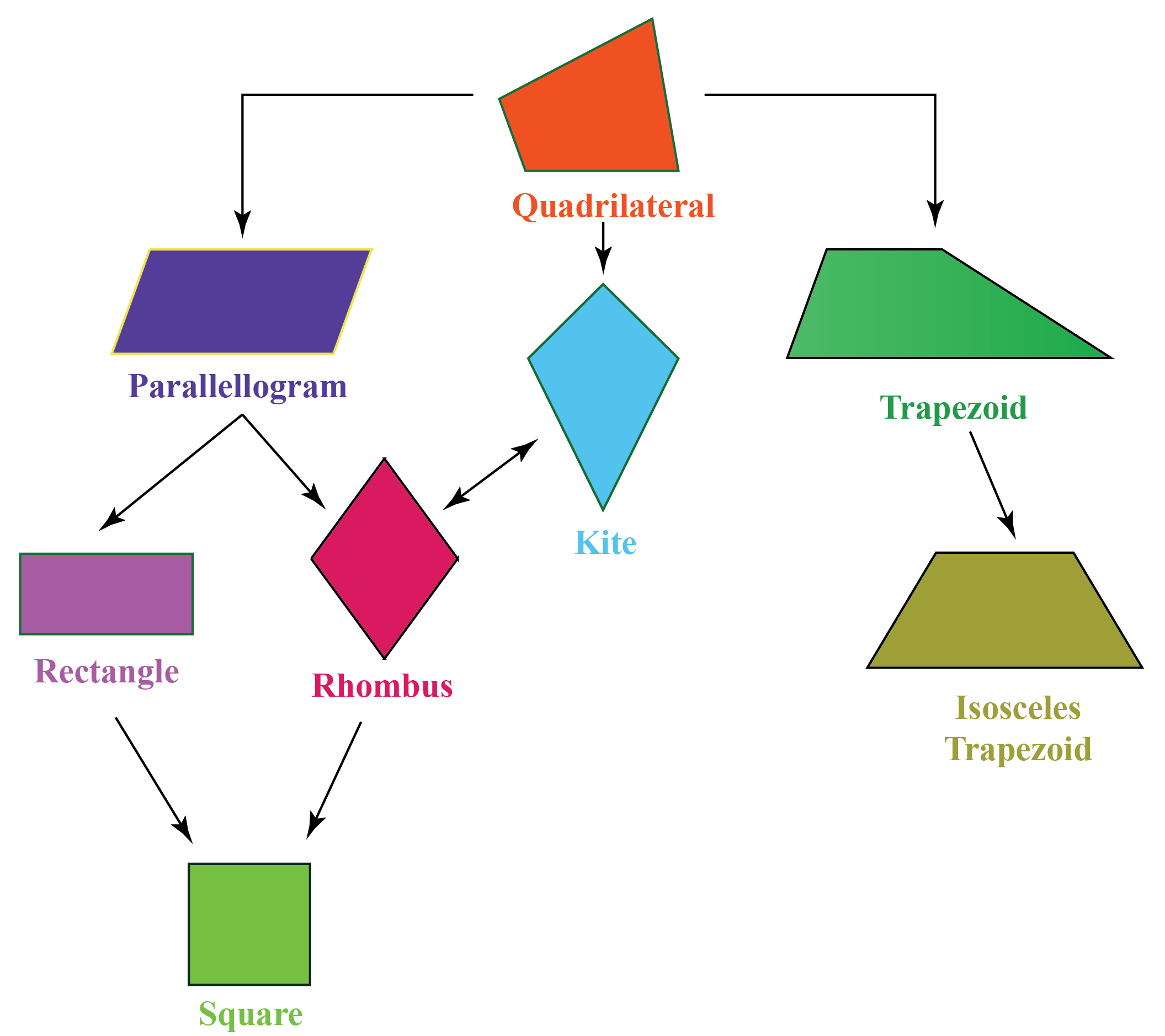From the above figure, we can note certain key points. For example:

• All squares are rectangles, but not all rectangles are squares.
• All squares are rhombuses, but not all rhombuses are squares.Think Tank
1. Can a kite be called a parallelogram?
2. What elements of a trapezium should be changed to make it a parallelogram?

### Illustration to Understand Properties of Quadrilaterals

Here is an illustration to understand the properties of quadrilaterals.

In this, you can select different types of quadrilaterals.

Then you can drag any of the vertices in "Red" and see how the angles and sides are changing but still, the properties are holding.

Help your child score higher with Cuemath’s proprietary FREE Diagnostic Test. Get access to detailed reports, customised learning plans, and a FREE counselling session. Attempt the test now.

### Common Mistakes​​​​​​

1. Any four-sided figure is a quadrilateral.

For example, the figure given below is a quadrilateral.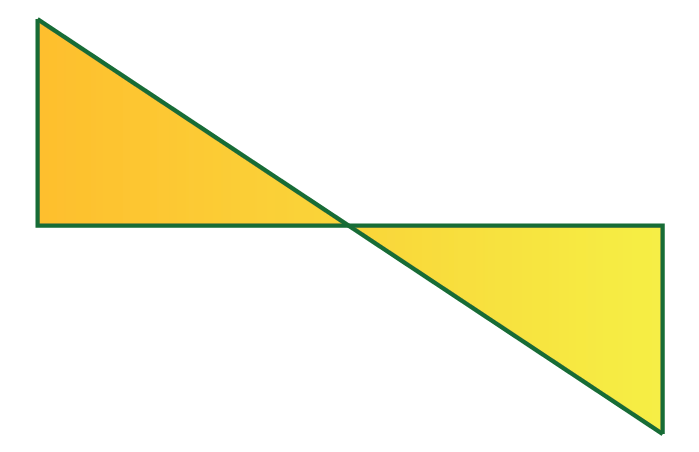Though the above figure has 4 sides and is a closed figure, it is NOT a quadrilateral.

2. All rectangles are squares.

Many rectangles do not have 4 equal sides and 4 equal angles. Therefore, all rectangles are NOT squares.

However, all squares are rectangles. As they all have 4 right angles, their opposite sides are parallel to each other and the opposite sides are equal to each other.

3. All rhombuses are squares.

Many rhombuses do not have four equal sides and four equal angles. Thus, all rhombuses are NOT squares.

However, all squares are rhombuses. As they have 4 equal sides, their opposite sides are parallel to each other.

The area of a quadrilateral is the number of unit squares that can be fit into it.

The following table lists the formulas for finding the area of quadrilaterals.

Square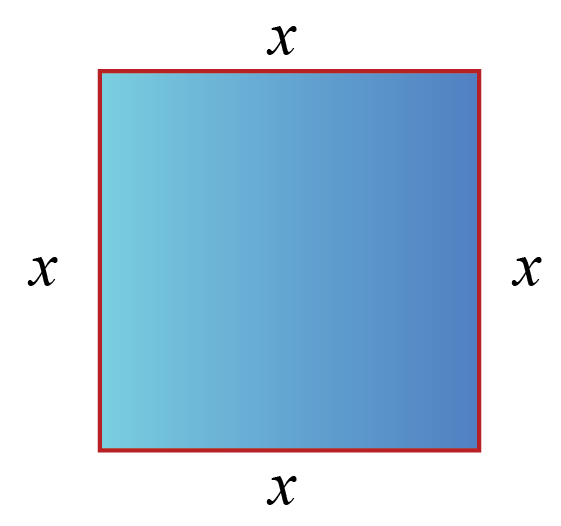$$x \times x$$
Rectangle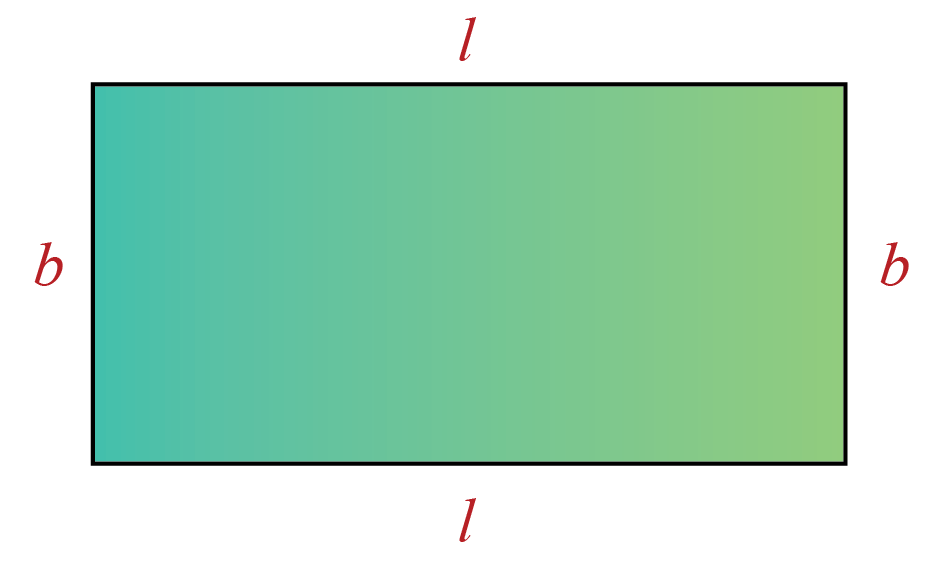$$l \times b$$
Parallelogram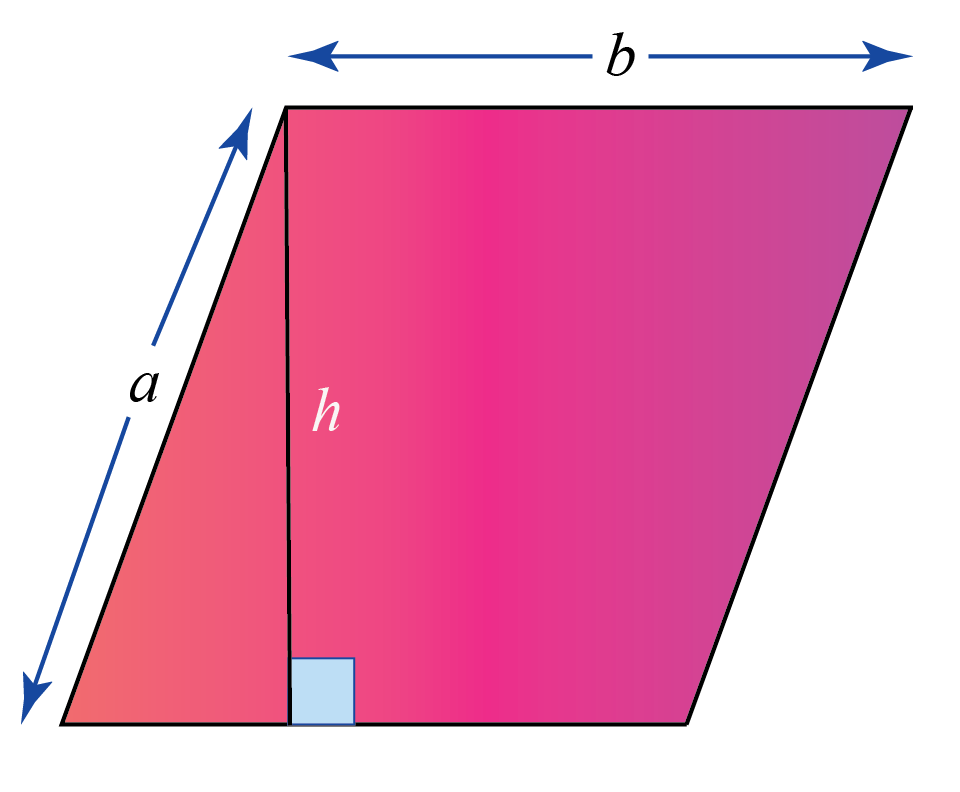$$b \times h$$
Isosceles Trapezium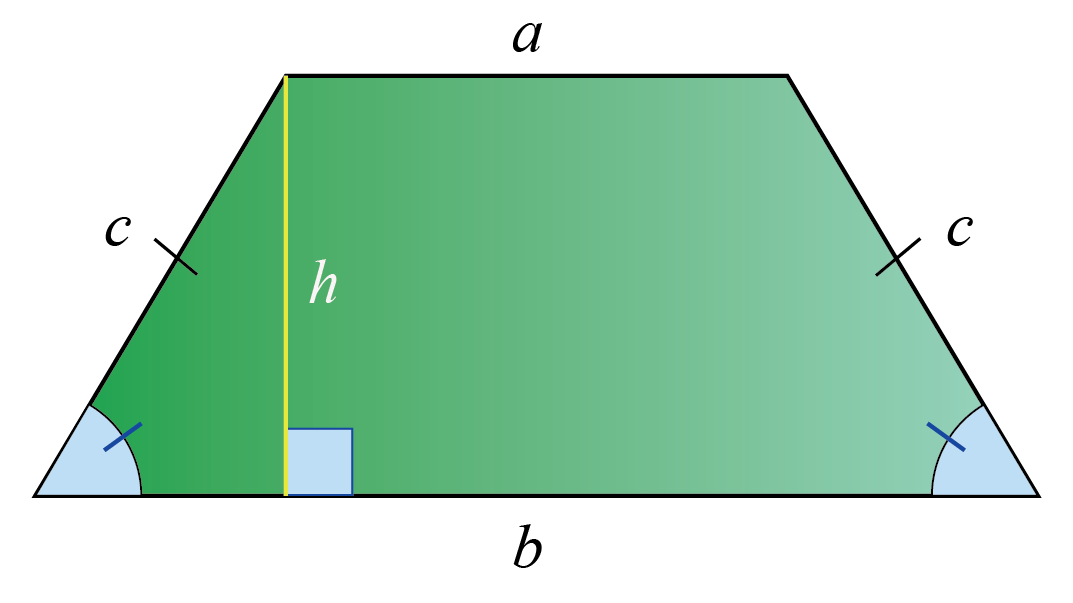$$\frac{1}{2}(a+b)h$$
Rhombus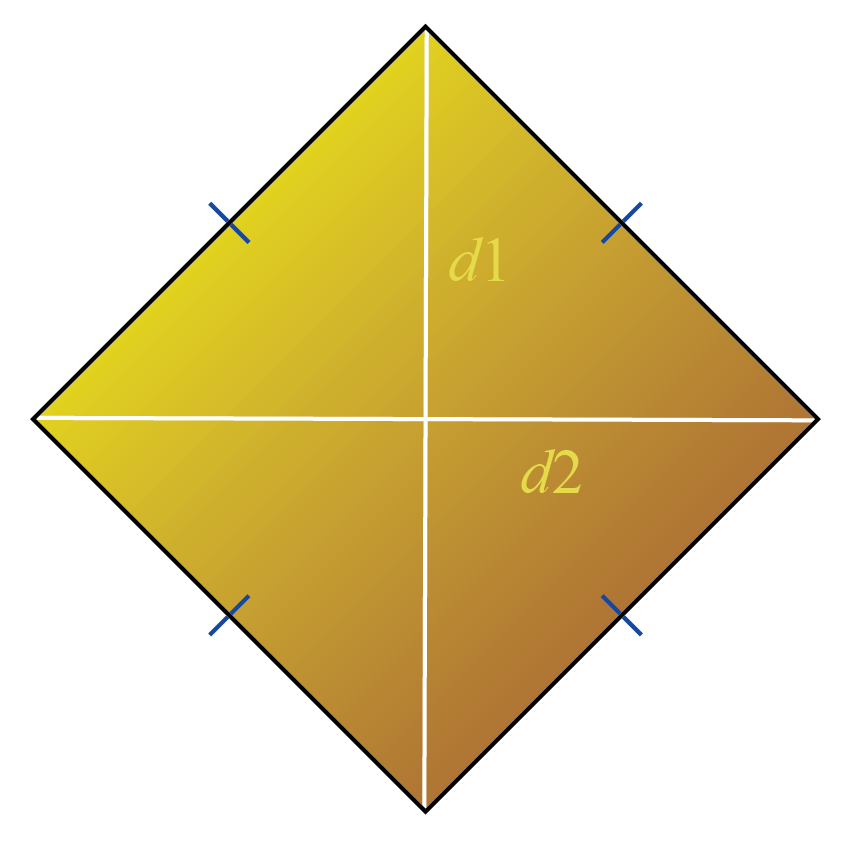$$\frac{1}{2} \times d_1 \times d_2$$
Kite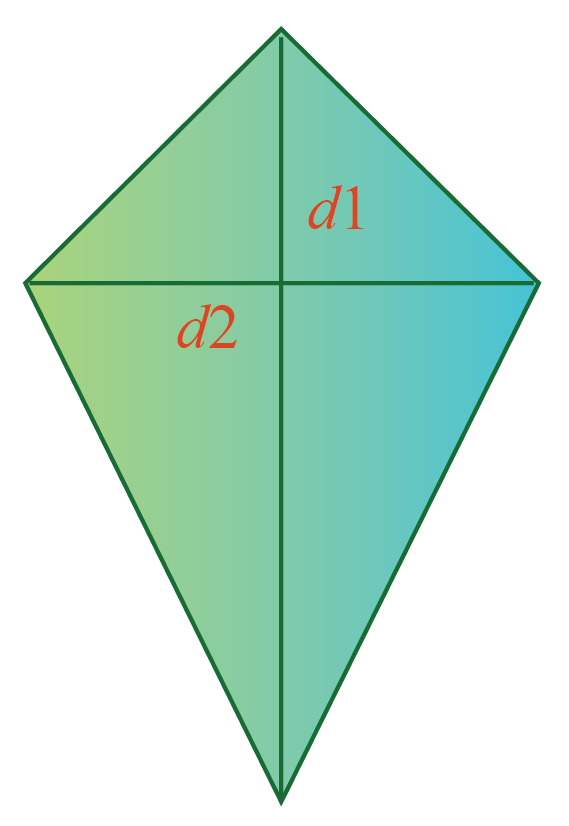$$\frac{1}{2} \times d_1 \times d_2$$

## Learn These Along with Quadrilateral!

Here are a few more lessons related to quadrilaterals.

These topics will not only help you master the concept for quadrilaterals, but also other topics related to it.

 Check out other related topics. Click on any of the short lessons you want to explore. Geometric Shapes Triangles Coordinate Geometry Euclid's Geometry Circles Solid Shapes Angles Parallelograms Angle sum property in quadrilaterals Heron's Formula and Quadrilaterals

 Example 1

Find the angle $$x^\circ$$ in the following figure.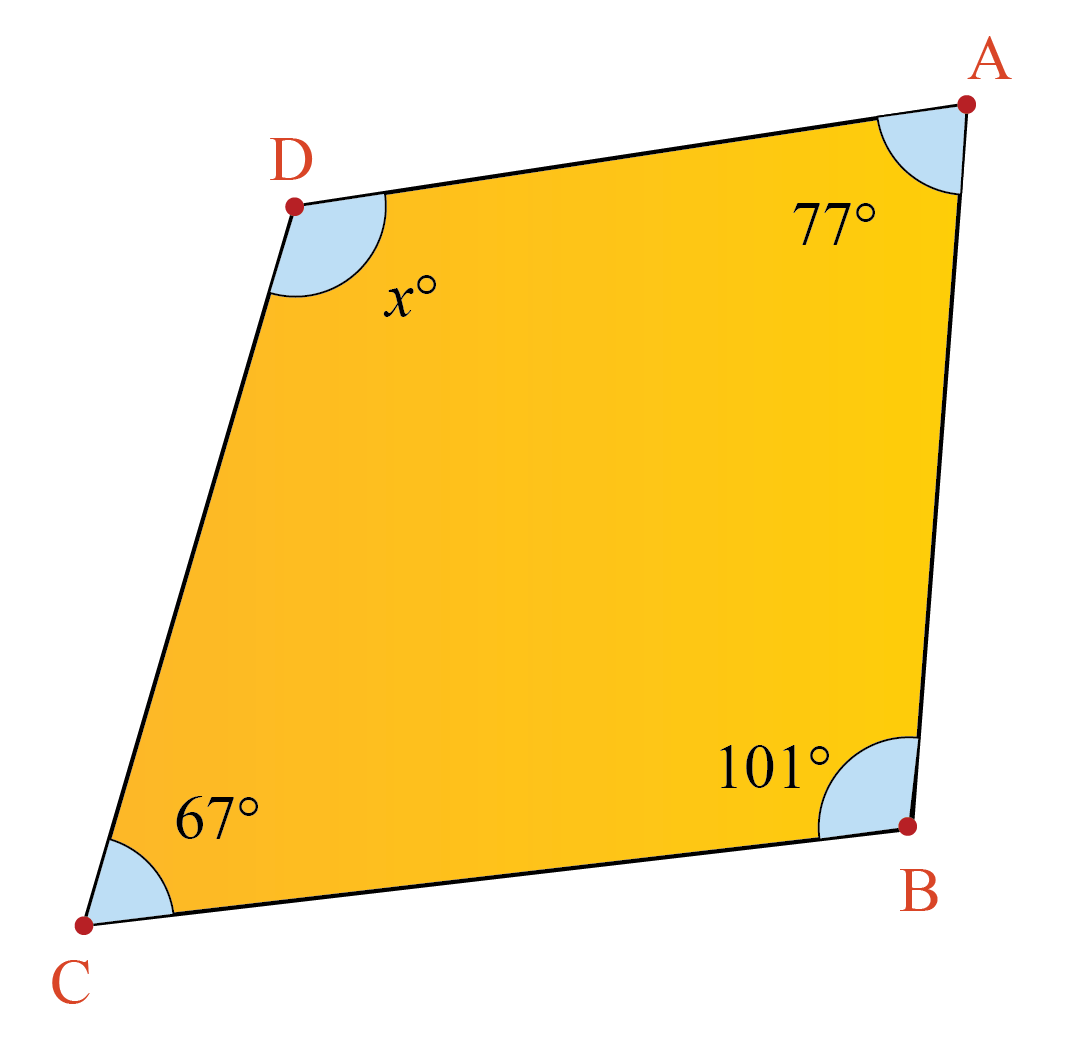Solution:

We know that the sum of the angles in a quadrilateral is $$360^\circ$$.

From the given figure, we get:

\begin{align} x+67+77+101 &=360\\[0.3cm] x+245&=360\\[0.3cm] x &=115 \end{align}

 $$\therefore x^\circ = 115^\circ$$
 Example 2

What is the area of a kite whose diagonals are of length $$10$$ cm and $$20$$ cm?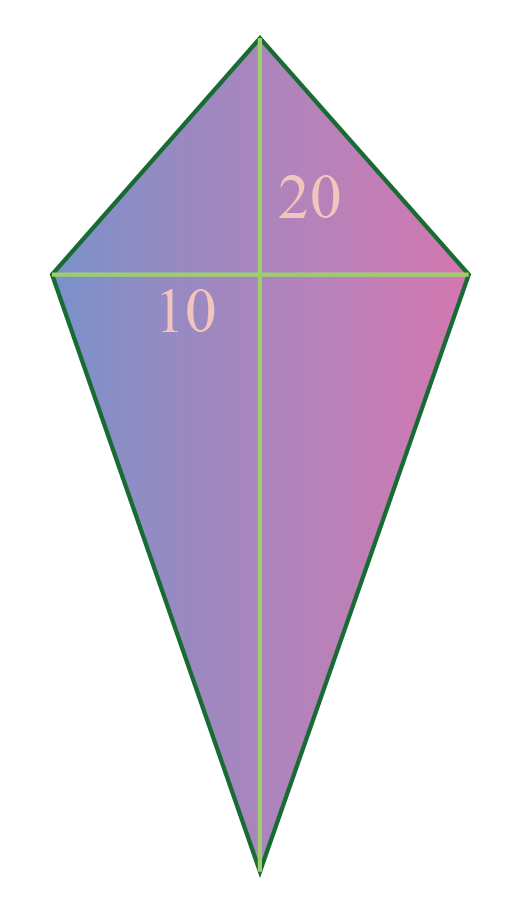Solution:

The diagonals of the given kite are:

$d_1=10\\d_2=20$

The area of the kite is calculated using the formula:

\begin{align} A &= \dfrac{1}{2} \times d_1 \times d_2\\[0.3cm] &= \dfrac{1}{2}\times 10 \times 20\\[0.3cm] &= 100 \end{align}

 $$\therefore$$ Area of the kite $$= 100 \text{ cm}^2$$
 Example 3

What is the area of the following isosceles trapezium?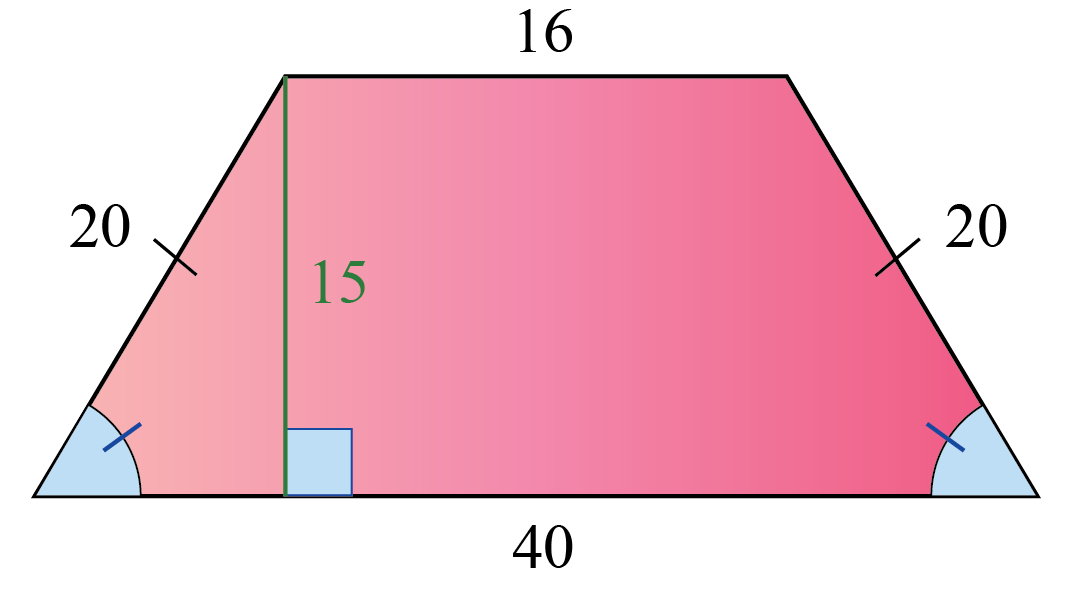Solution:

The area of an isosceles trapezium is calculated using the formula:

Area of an isosceles trapezium \begin{align}=\frac{1}{2}(a+b)h\end{align}

In this example, the bases of the given isosceles trapezium are:

$a=16\\b=40$

We can calculate the area of trapezium using the above formula as follows:

\begin{align} \!&=\frac{1}{2}(16+40)15\\[0.3cm] \!&=\frac{1}{2}(840)\\[0.3cm] \!&=420\end{align}

 $$\therefore$$ Area of the trapezium $$=420\text{ unit}^2$$Challenging Questions
1. In which of the following quadrilateral are the diagonals equal?
a) Isosceles Trapezium
b) Rectangle
c) Square
d) Rhombus
2. In which of the following quadrilateral do the diagonals bisect each other?
a) Trapezium
b) Parallelogram
c) Square
d) Isosceles Trapezium

Here are a few activities for you to practice. Select/Type your answer and click the "Check Answer" button to see the result.

## Summary

The magic of math lies in the amazing concepts that it is built upon. At Cuemath, we explore and interact with these concepts in a fun way!

The math journey around quadrilaterals starts with what a student already knows, and goes on to creatively crafting a fresh concept in the young minds. Done in a way that not only it is relatable and easy to grasp, but also will stay with them forever.

The best part, this isn’t the end. With a universe built around quadrilaterals at Cuemath, one can take their math journey forward with solved examples, practice questions, quizzes, worksheets, practice papers, and so much more. Again, all of it, exclusively around quadrilaterals.

## 1. What are the various types of quadrilaterals?

The different types of quadrilaterals are:

1. Square
2. Rectangle
3. Parallelogram
4. Trapezium
5. Rhombus
6. Kite

## 2. Are all rhombuses also squares?

No, all rhombuses are NOT squares as they may not have four right angles.

However, all squares are rhombuses as all their sides are equal in length.

## 3. Are all rectangles also parallelograms?

Yes, all rectangles are parallelograms as they have two pairs of parallel opposite sides.

## 4. Are all rhombuses also kites?

Yes, every rhombus is a kite as they have two pairs of equal adjacent sides.

grade 9 | Questions Set 1
grade 9 | Questions Set 2
grade 9 | Questions Set 1